﻿ 基于多粒度结构的网络表示学习
«上一篇文章快速检索 高级检索

 智能系统学报2019, Vol. 14Issue (6): 1233-1242  DOI: 10.11992/tis.2019050450

### 引用本文ZHANG Lei, QIAN Feng, ZHAO Shu, et al. Network representation learning based on multi-granularity structure[J]. CAAI Transactions on Intelligent Systems, 2019, 14(6): 1233-1242. DOI: 10.11992/tis.201905045.### 文章历史

1. 安徽大学 计算机科学与技术学院，安徽 合肥 230601;
2. 铜陵学院 数学与计算机学院，安徽 铜陵 244061

Network representation learning based on multi-granularity structure
ZHANG Lei 1,2, QIAN Feng 1,2, ZHAO Shu 1, CHEN Jie 1, ZHANG Yanping 1, LIU Feng 1
1. School of Computer Science and Technology, Anhui University, Hefei 230601, China;
2. School of Mathematics and Computer Science, Tongling University, Tongling 244061, China
Abstract: The Graph Convolution Network (GCN) can adapt to graphs with different structures. However, most GCN-based models have difficulty effectively capturing the high-order similarity of the network. Simply adding a convolution layer will cause the output features to be too smooth and difficult to distinguish. Moreover, the deep neural network is more difficult to train. In this paper, multi-granularity structure and a GCN are combined to represent the node characteristics of the learning network. A multi-granularity structure-based network representation learning method, Multi-GS, is proposed. First, based on the idea of modularity clustering and granular computing, hierarchical multi-granularity space was used to replace the original single-layer network topology space. The GCN model was then used to learn the representation of granules in different coarse- and fine-granularity spaces. Finally, representations of the different grains were combined into representations of nodes in the original space from coarse to fine. Experimental results showed that multi-GS can capture a variety of structural information, including first-order and second-order similarity, intra-community similarity (high-order structure), and inter-community similarity (global structure). In most cases, using multi-granularity structure can improve the classification performance of node classification tasks.
Key words: network represent learning    network topology    modularity increment    network coarsening    multi-granularity structure    Graph Convolution Network    node classification    link prediction

1 问题定义

 $Q = \frac{1}{{2m}}\sum\limits_{c \in C} {\left( {\frac{{l_c}}{{2m}} - {{\left( {\frac{{D_c}}{{2m}}} \right)}^2}} \right)}$ (1)

 $\begin{gathered} \Delta Q_{pq} = Q_k - Q_p - Q_q = \\ \displaystyle \frac{{{{\left( {l_p + l_q} \right)}^2}}}{{2m}} - {\left( {\frac{{D_p + D_q}}{{2m}}} \right)^2} - \frac{{l_p}}{{2m}} + {\left( {\frac{{D_p}}{{2m}}} \right)^2} - \\ \displaystyle \frac{{l_q}}{{2m}} + {\left( {\frac{{D_q}}{{2m}}} \right)^2} = \frac{{l_p}}{{2m}} + \frac{{l_{pq}}}{m} + \frac{{l_q}}{{2m}} - {\left( {\frac{{D_p}}{{2m}}} \right)^2} - \\ \displaystyle \frac{{D_pD_q}}{{2{m^2}}} - {\left( {\frac{{D_q}}{{2m}}} \right)^2} - \frac{{l_p}}{{2m}} + {\left( {\frac{{D_p}}{{2m}}} \right)^2} - \frac{{l_q}}{{2m}} + {\left( {\frac{{D_q}}{{2m}}} \right)^2} = \\ \displaystyle \frac{{l_{pq}}}{m} - \frac{{D_pD_q}}{{2{m^2}}} \\ \end{gathered}$ (2)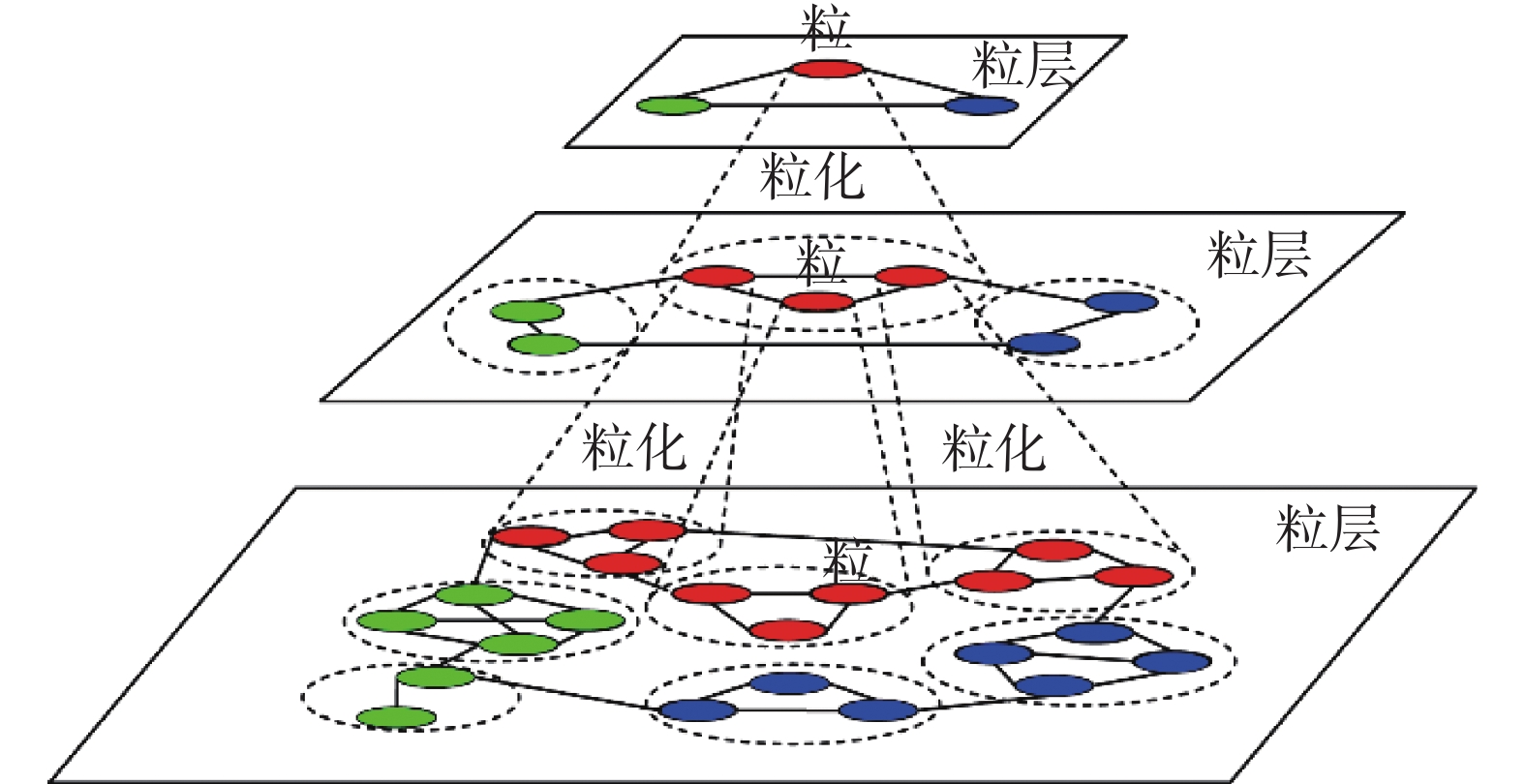Download: 图 1 网络拓扑的粒化分层示例 Fig. 1 An example of hierarchical view of network topology

2 网络表示学习方法Multi-GS

Multi-GS首先基于模块度构建多粒度的网络分层结构；接着使用GCN模型依次学习不同粒层中所有粒的特征向量；然后自底向上逐层对粒的特征向量进行映射、拼接；最后输出最终的结果作为初始网络中节点的特征表示，如图2所示。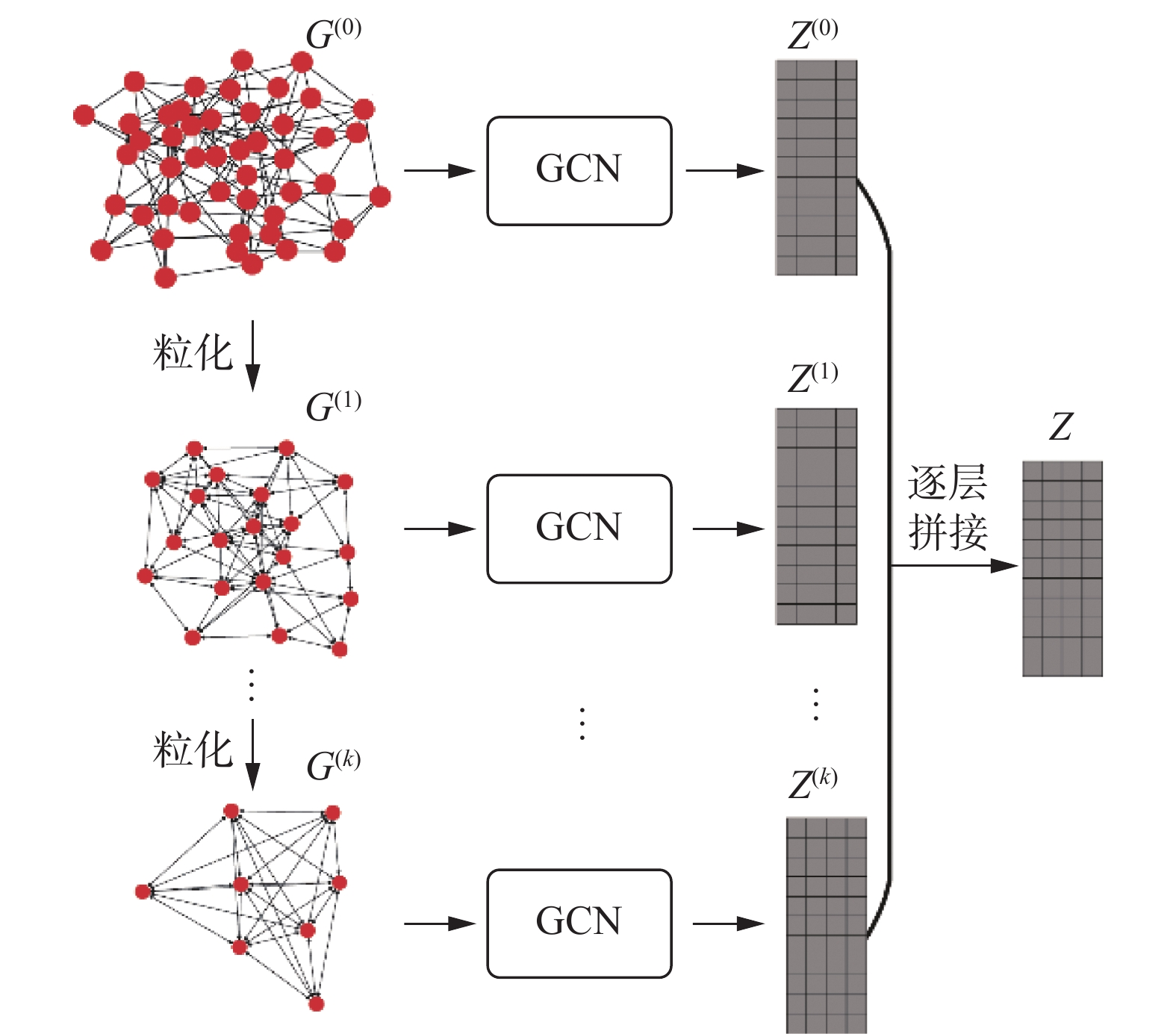Download: 图 2 Multi-GS方法的框架 Fig. 2 Framework of Multi-GS approach
2.1 基于模块度的网络粒化分层

1)level = 0；

2)granuleGraph = copy_Graph(G)；/*复制图结构*/

3)Qnew=modularity()；/*按式(1)计算模块度*/

4)repeat

5) ${\rm{Gr}}{^{\left( {\rm level} \right)}}\leftarrow {\rm{add_Graph}}({\rm granuleGraph})$

6) $C \leftarrow \left\{ {{C_i}| {{v_i} \in {\rm granuleGraph}}} \right\}$

7)repeat

8)Qcur= Qnew

9)for each vi in granuleGraph do

10)将粒 ${v_i}$ 从自身所在的集合中移出；

11)for ${v_j}$ in $\Gamma ({v_i})$ do

12)按式(2)计算粒 ${v_i}$ 移入集合 ${C_j}$ 后的模块度增量 $\Delta Q$

13)end for

14)将粒 ${v_i}$ 并入max(∆Q)>0的粒集合；

15)end for

16)Qnew=modularity()；

17)untill (Qcur = Qnew)

18) ${V^*} \leftarrow \left\{ {v_i^*|{\rm{each}}\;{C_i}\;{\rm{in}}\;{\rm granuleGraph}} \right\}$

19) ${E^*} \leftarrow \left\{ {e_{ij}^*|{e_{ij}},{v_i} \in {C_i},{v_j} \in {C_j}\;{\rm{and}}\;{C_i} \ne {C_j}} \right\}$

20) $\displaystyle{W^*} \leftarrow \left\{ {W_{ij}^*|\sum {{w_{ij}}} ,\;{\rm{if}}\;{v_i} \in {C_i}\;{\rm{and}}\;{v_j} \in {C_j}} \right\}$

21) ${\rm granuleGraph} \leftarrow {\rm{Graph}}\left( {{V^*},{E^*},{W^*}} \right)$

22)level = level +1；

23)untill (level>k)

24)return ${\rm{Gr}}{^{(0)}},{\rm{Gr}}{^{(1)}},\cdots,{\rm{Gr}}{^{(k)}}$

2.2 基于图卷积网络的粒特征表示学习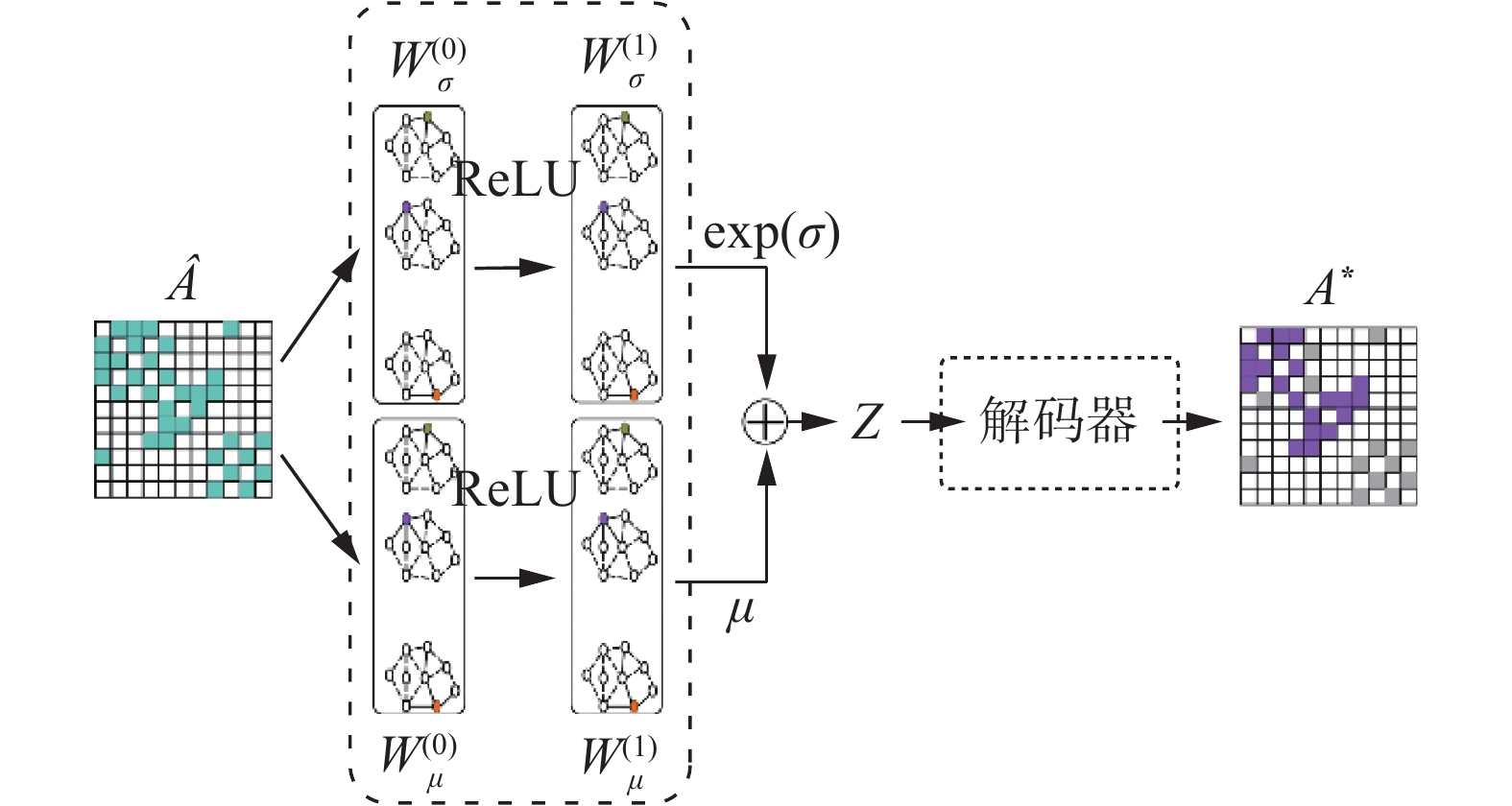Download: 图 3 图卷积神经网络模型结构 Fig. 3 The structure of GCN model

GCN模型的输入是不同分层中的粒关系矩阵 ${{A}}$ ${{A}} \in {{\bf{R}}^{N \times N}}$ 表示同一粒层中粒间的连接关系，其中， $N$ 表示粒的数量。给定粒 $i$ 和粒 $j$ ，则 $A_{ij} = w_{ij}$ $w_{ij}$ 表示粒 $i$ 和粒 $j$ 间的连接权重。首先，利用式(3)计算得到归一化的矩阵 $\widehat {{A}} \in {{\bf{R}}^{N \times N}}$

 $\widehat {{A}} = {{{D}}^{ - \frac{1}{2}}}\widetilde {{A}}{{{D}}^{ - \frac{1}{2}}}$ (3)

 $\mu = f\left( {\widehat {{A}}f\left( {\widehat {{A}}{{W}}_\mu ^{\left( 0 \right)}} \right){{W}}_\mu ^{\left( 1 \right)}} \right)$ (4)
 $\sigma = f\left( {\widehat {{A}}f\left( {\widehat {{A}}{{W}}_\sigma ^{\left( 0 \right)}} \right){{W}}_\sigma ^{\left( 1 \right)}} \right)$ (5)
 ${{Z}} \sim {\cal{N}}\left( {\mu ,\exp (\sigma )} \right)$ (6)
 ${{{A}}^ * } = {\rm{sigmoid}}\left( {{{Z}} * {{{Z}}^{\rm{T}}}} \right)$ (7)

 ${{\cal{L}}_{\rm latent}} = - \left( {\frac{{0.5}}{N}} \right) \cdot {\rm{mean}}\left( {\sum\limits_{i = 1}^d {\left( {1 + 2 \log \left( \sigma \right) - {\mu ^2} - {\sigma ^2}} \right)} } \right)$ (8)

 $\begin{gathered} {\rm{Loss}} = {{A}} \cdot \left[ - \log \left( {{\rm{sigmoid}}\left( {{{{A}}^ * }} \right)} \right) * {{{W}}^{(1)}}\right] + \\ \left( {1 - {{A}}} \right) \cdot \left[ - \log \left( {1 - {\rm{sigmoid}}\left( {{{{A}}^ * }} \right)} \right) \right] \\ \end{gathered}$ (9)
 ${{{W}}^{(1)}} = {{\left( {N \cdot N - \sum\limits_{i = 1}^N {{A_i}} } \right)} \Big/ {\sum\limits_{i = 1}^N {{A_i}} }}$ (10)
 ${{{W}}^{(2)}} = {{N \cdot N} \Big/ {\left( {N \cdot N - \sum\limits_{i = 1}^N {{A_i}} } \right)}}$ (11)
 ${{\cal{L}}_{\rm reconst}} = {{{W}}^{(2)}}{\rm{mean}}\left( {{\rm{Loss}}\left( {{{A}},{{{A}}^ * },{{{W}}^{(1)}}} \right)} \right)$ (12)

 ${\cal{L}} = {{\cal{L}}_{\rm latent}} + {{\cal{L}}_{\rm reconst}}$ (13)
2.3 算法描述

1) ${\rm{Gr}}{^{(0)}},{\rm{Gr}}{^{(1)}},\cdots,{\rm{Gr}}{^{(k)}}\leftarrow {\rm{Graphgranular}}(G)$

2)for m = 0 to k do

3) ${{{Z}}^{\left( {\rm{m}} \right)}} \leftarrow {\rm{GCN}}\left( {{\rm{Gr}}{^{\left( {{m}} \right)}}.{{A}},\Theta } \right)$

4)end for

5)for n = k to 1 do

6) ${{{Z}}^{\left( {{n}} \right)}} \leftarrow {\rm{projection}}\left( {{{{Z}}^{\left( {{n}} \right)}}} \right)$

7) ${{{Z}}^{\left( {{{n}} - 1} \right)}} \leftarrow {\rm{concatenate}}\left( {{{{Z}}^{\left( {{n}} \right)}},{{{Z}}^{\left( {{{n}} - 1} \right)}}} \right)$

8)end for

9)ZZ(0)

10)return Z

3 实验和结果分析

3.1 实验设定

1) 数据集。表 1 数据集信息 Tab.1 Datasets information

2) 对比算法。

DeepWalk：使用随机游走获取节点序列，通过SkipGram方法学习节点表示。

Node2Vec：类似于DeepWalk，但是使用有偏向的随机游走获取节点序列。

GraRep：通过构造 $k$ 步概率转移矩阵学习节点表示，能够保留节点的高阶相似性。

DNGR：使用随机冲浪方法获取节点的高阶相似性，利用深度神经网络学习节点表示。

3) 参数设定。

3.2 节点分类表 2 Citeseer数据集上的Micro-F1和Macro-F1结果 Tab.2 Micro-F1 and Macro-F1 results on Citeseer dataset表 3 PPI数据集上的Micro-F1和Macro-F1结果 Tab.3 Micro-F1 and Macro-F1 results on PPI dataset表 4 WiKi数据集上的Micro-F1和Macro-F1结果 Tab.4 Micro-F1 and Macro-F1 results on WiKi dataset表 5 BlogCatalog数据集上的Micro-F1和Macro-F1结果 Tab.5 Micro-F1 and Macro-F1 results on BlogCatalog dataset

3.3 链接预测表 6 链接预测任务中不同数据集上的AUC结果 Tab.6 AUC score on all datasets

3.4 可视化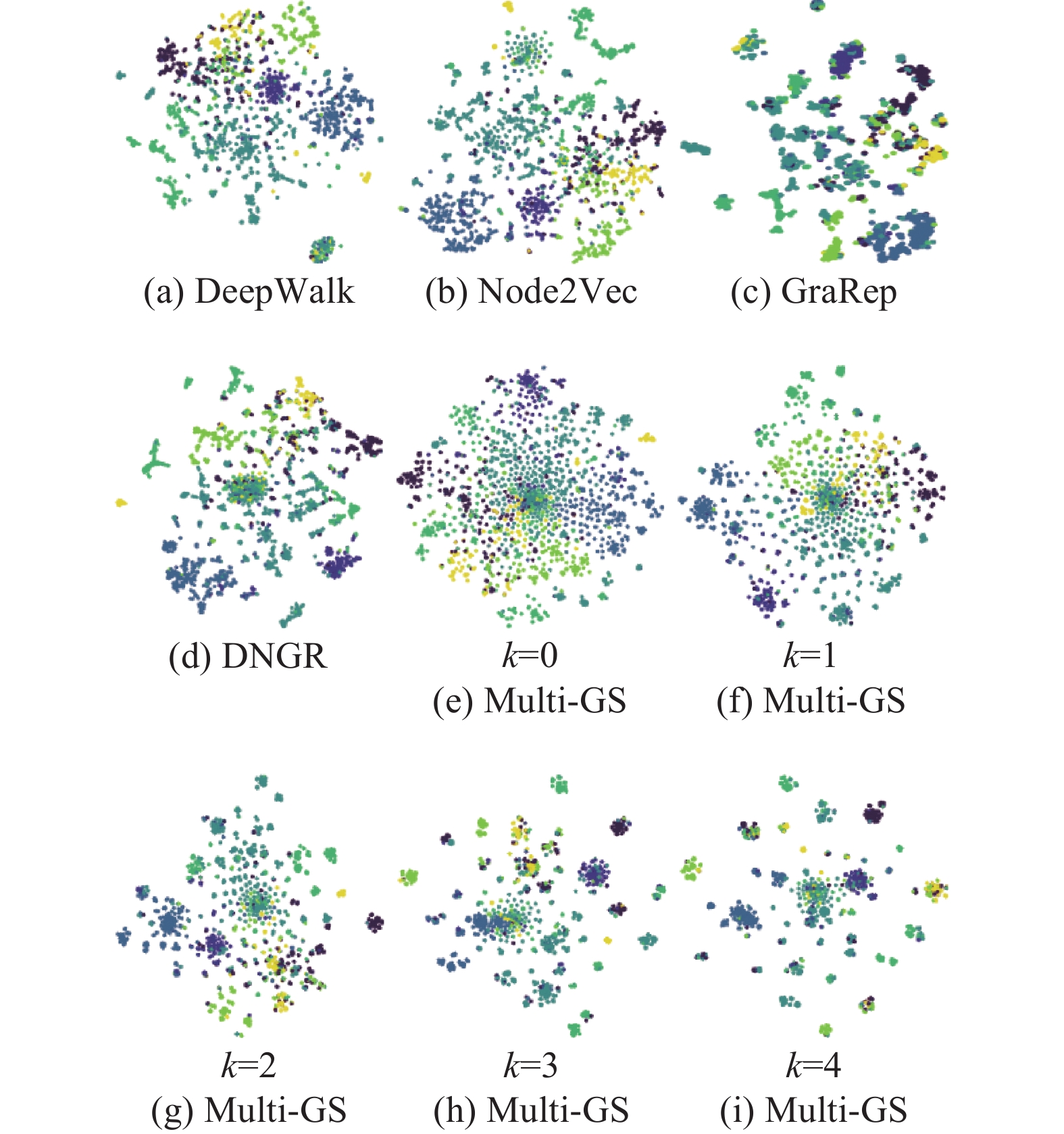Download: 图 4 Cora数据集的可视化结果 Fig. 4 The visualization of Cora dataset

3.5 参数敏感性分析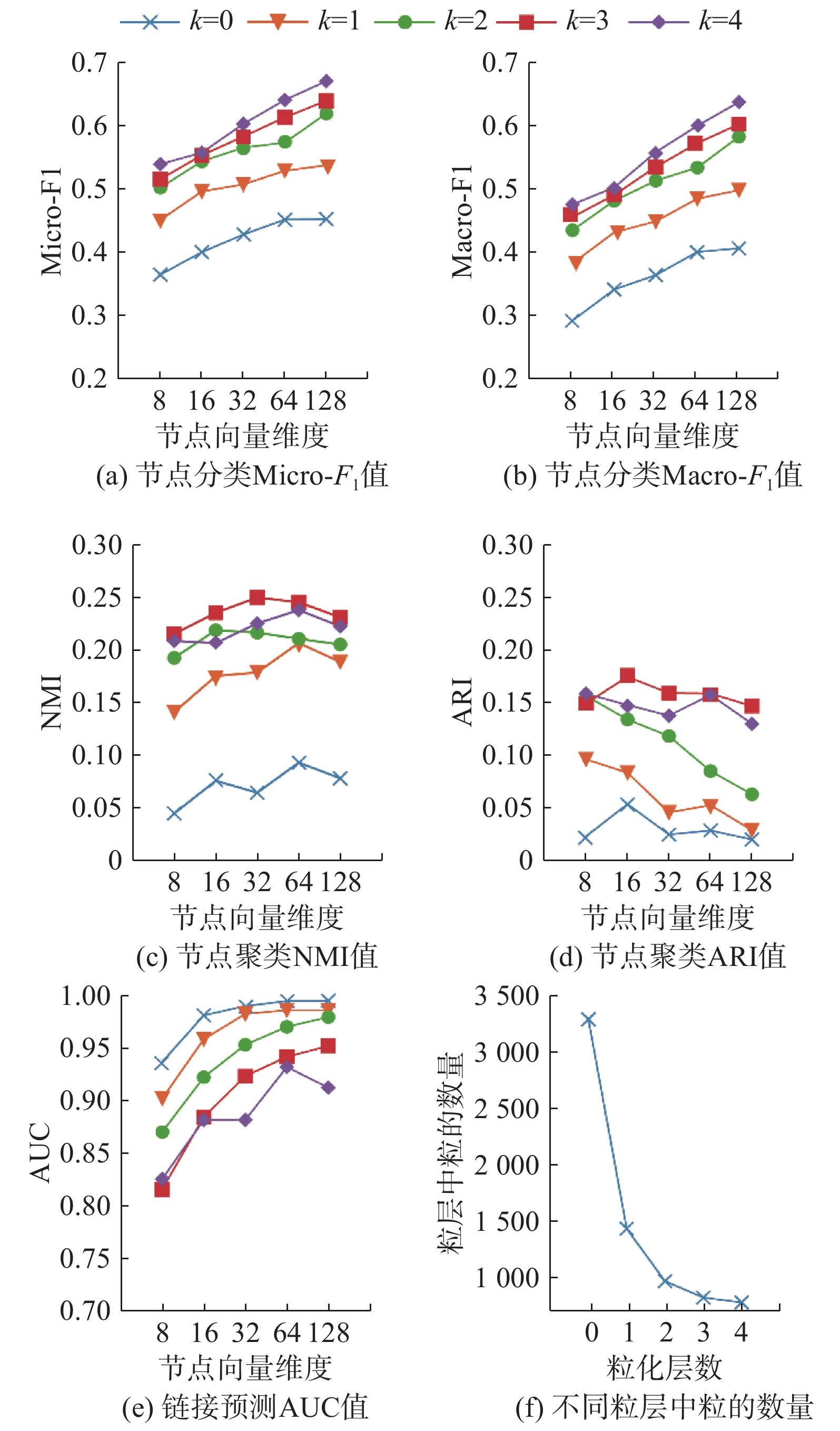Download: 图 5 参数敏感性分析 Fig. 5 Parametric sensitivity analysis

4 结束语

  涂存超, 杨成, 刘知远, 等. 网络表示学习综述[J]. 中国科学: 信息科学, 2017, 47(8): 980-996. TU Cunchao, YANG Cheng, LIU Zhiyuan, et al. Network representation learning: an overview[J]. Scientia sinica informationis, 2017, 47(8): 980-996. (0)  SHEIKH N, KEFATO Z T, MONTRESOR M. Semi-supervised heterogeneous information network embedding for node classification using 1D-CNN[C]//Proceedings of the Fifth International Conference on Social Networks Analysis, Management and Security. Valencia, Spain, 2018: 177–181. (0)  XU Guangluan, WANG Xiaoke, WANG Yang, et al. Edge-nodes representation neural machine for link prediction[J]. Algorithms, 2019, 12(1): 12. DOI:10.3390/a12010012 (0)  HU Xuegang, HE Wei, LI Lei, et al. An efficient and fast algorithm for community detection based on node role analysis[J]. International journal of machine learning and cybernetics, 2019, 10(4): 641-654. DOI:10.1007/s13042-017-0745-x (0)  PEREDA M, ESTRADA E. Visualization and machine learning analysis of complex networks in hyperspherical space[J]. Pattern recognition, 2019, 86: 320-331. DOI:10.1016/j.patcog.2018.09.018 (0)  SHI Chuan, HU Binbin, ZHAO W X, et al. Heterogeneous information network embedding for recommendation[J]. IEEE transactions on knowledge and data engineering, 2019, 31(2): 357-370. DOI:10.1109/TKDE.2018.2833443 (0)  PEROZZI B, AL-RFOU R, SKIENA S. DeepWalk: online learning of social representations[C]//Proceedings of the 20th ACM SIGKDD International Conference on Knowledge Discovery and Data Mining. New York, USA, 2014: 701–710. (0)  GROVER A, LESKOVEC J. node2vec: Scalable feature learning for networks[C]//Proceedings of the 22th ACM SIGKDD International Conference on Knowledge Discovery and Data Mining. San Francisco, California, USA, 2016: 855–864. (0)  CAO Shaosheng, LU Wei, XU Qiongkai. GraRep: learning graph representations with global structural information[C]// Proceedings of the 24th ACM International on Conference on Information and Knowledge Management. Melbourne, Australia, 2015: 891–900. (0)  PEROZZI B, KULKARNI V, CHEN Haochen, et al. Don't walk, Skip!: Online learning of multi-scale network embeddings[C]//Proceedings of the 2017 IEEE/ACM International Conference on Advances in Social Networks Analysis and Mining 2017. Sydney, Australia, 2017: 258–265. (0)  CAO Shaosheng, LU Wei, XU Qiongkai. Deep neural networks for learning graph representations[C]//Proceedings of the 30th AAAI Conference on Artificial Intelligence. Phoenix, USA, 2016: 1145–1152. (0)  RIBEIRO L F R, SAVERESE P H P, FIGUEIREDO D R. struc2vec: Learning node representations from structural identity[C]//Proceedings of the 23rd ACM SIGKDD International Conference on Knowledge Discovery and Data Mining. Halifax, Canada, 2017: 385–394. (0)  WANG Xiao, CUI Peng, WANG Jing, et al. Community preserving network embedding[C]//Proceedings of the 31th AAAI Conference on Artificial Intelligence. San Francisco, California, USA, 2017: 203–209. (0)  方莲娣, 张燕平, 陈洁, 等. 基于三支决策的非重叠社团划分[J]. 智能系统学报, 2017, 12(3): 293-300. FANG Liandi, ZHANG Yanping, CHEN Jie, et al. Three-way decision based on non-overlapping community division[J]. CAAI transactions on intelligent systems, 2017, 12(3): 293-300. (0)  DONNAT C, ZITNIK M, HALLAC D, et al. Learning structural node embeddings via diffusion wavelets[C]//Proceedings of the 24th ACM SIGKDD International Conference on Knowledge Discovery & Data Mining. London, UK, 2018: 1320–1329. (0)  CHEN Haochen, PEROZZI B, HU Hifan, et al. HARP: hierarchical representation learning for networks[C]// Proceedings of the 32th AAAI Conference on Artificial Intelligence. New Orleans, Louisiana, USA, 2018: 2127–2134. (0)  ZHANG Si, TONG Hanghang, XU Jiejun, et al. Graph convolutional networks: algorithms, applications and open challenges[C]//Proceedings of the 7th International Conference on Computational Data and Social Networks. Shanghai, China, 2018: 79–91. (0)  KIPF T N, WELLING M. Semi-supervised classification with graph convolutional networks[C/OL]. [2019-01-28]. https://arxiv.org/pdf/1609.02907.pdf. (0)  LI Qimai, HAN Zhichao, WU Xiaoming. Deeper insights into graph convolutional networks for semi-supervised learning[C]//Proceedings of the 32th AAAI Conference on Artificial Intelligence. New Orleans, Louisiana, USA, 2018: 3538–3545. (0)  张燕平, 张铃, 吴涛. 不同粒度世界的描述法——商空间法[J]. 计算机学报, 2004, 27(3): 328-333. ZHANG Yanping, ZHANG Ling, WU Tao. The representation of different granular worlds: a quotient space[J]. Chinese journal of computers, 2004, 27(3): 328-333. DOI:10.3321/j.issn:0254-4164.2004.03.006 (0)  赵姝, 柯望, 陈洁, 等. 基于聚类粒化的社团发现算法[J]. 计算机应用, 2014, 34(10): 2812-2815. ZHAO Shu, KE Wang, CHEN Jie, et al. Community detection algorithm based on clustering granulation[J]. Journal of computer applications, 2014, 34(10): 2812-2815. DOI:10.11772/j.issn.1001-9081.2014.10.2812 (0)  NEWMAN M E J. Fast algorithm for detecting community structure in networks[J]. Physical review E, 2003, 69(6): 066133. (0)  赵姝, 赵晖, 陈洁, 等. 基于社团结构的多粒度结构洞占据者发现及分析[J]. 智能系统学报, 2016, 11(3): 343-351. ZHAO Shu, ZHAO Hui, CHEN Jie, et al. Recognition and analysis of structural hole spanner in multi-granularity based on community structure[J]. CAAI transactions on intelligent systems, 2016, 11(3): 343-351. (0)  KIPF T N, WELLING M. Variational graph auto-encoders[C/OL]. [2019-01-28]. https://arxiv.org/pdf/1611.07308.pdf. (0)  MCCALLUM A K, NIGAM K, RENNIE J, et al. Automating the construction of internet portals with machine learning[J]. Information retrieval, 2000, 3(2): 127-163. DOI:10.1023/A:1009953814988 (0)  BREITKREUTZ B J, STARK C, REGULY T, et al. The BioGRID interaction database: 2008 update[J]. Nucleic acids research, 2008, 36: D637-D640. (0)  GAO Hongchang, HUANG Heng. Self-paced network embedding[C]//Proceedings of the 24th ACM SIGKDD International Conference on Knowledge Discovery & Data Mining. London, UK, 2018: 1406–1415. (0)  TANG Lei, LIU Huan. Relational learning via latent social dimensions[C]//Proceedings of the 15th ACM SIGKDD International Conference on Knowledge Discovery and Data Mining. Paris, France, 2009: 817–826. (0)  FAWCETT T. An introduction to ROC analysis[J]. Pattern recognition letters, 2006, 27(8): 861-874. DOI:10.1016/j.patrec.2005.10.010 (0)  FAHAD A, ALSHATRI N, TARI Z, et al. A survey of clustering algorithms for big data: Taxonomy and empirical analysis[J]. IEEE transactions on emerging topics in computing, 2014, 2(3): 267-279. DOI:10.1109/TETC.2014.2330519 (0)